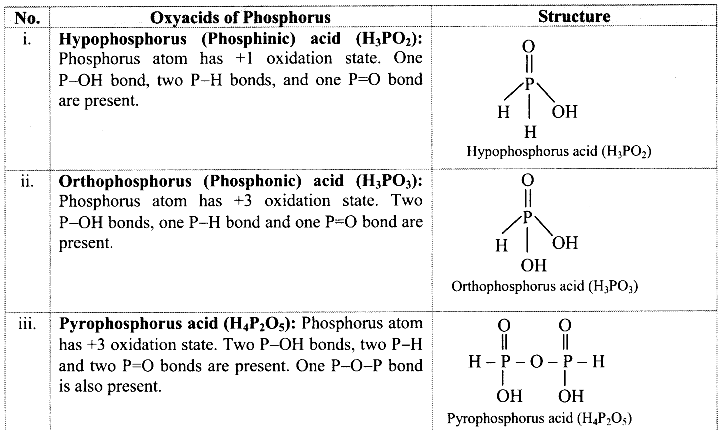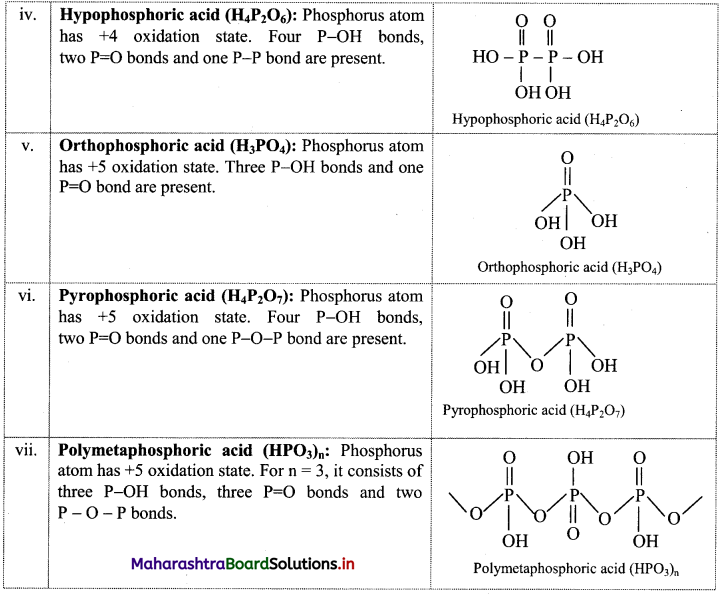# Maharashtra Board Class 11 Chemistry Solutions Chapter 9 Elements of Group 13, 14 and 15

Balbharti Maharashtra State Board 11th Chemistry Textbook Solutions Chapter 9 Elements of Group 13, 14 and 15 Textbook Exercise Questions and Answers.

## Maharashtra State Board 11th Chemistry Solutions Chapter 9 Elements of Group 13, 14 and 15

1. Choose the correct option.

Question A.
Which of the following is not an allotrope of carbon?
a. buckyball
b. diamond
c. graphite
d. emerald
d. emerald

Question B.
………… is inorganic graphite.
a. borax
b. diborane
c. boron nitride
d. colemanite
c. boron nitride

Question C.
Haber’s process is used for the preparation of ………….
a. HNO3
b. NH3
c. NH2CONH2
d. NH4OH
b. NH3

Question D.
Thallium shows a different oxidation state because ……………
a. of inert pair effect
b. it is an inner transition element
c. it is metal
d. of its high electronegativity
a. of inert pair effect

Question E.
Which of the following shows the most prominent inert pair effect?
a. C
b. Si
c. Ge
d. Pb
d. Pb2. Identify the group 14 element that best fits each of the following description.

A. Non-metallic element
B. Form the most acidic oxide
C. They prefer +2 oxidation state.
D. Forms strong π bonds.
i. Carbon (C)
ii. Carbon
iii. Tin (Sn) and lead (Pb)
iv. Carbon

3. Give reasons.

A. Ga3+ salts are better reducing agent while Tl3+ salts are better oxidising agent.
B. PbCl4 is less stable than PbCl2
A. i. Both gallium (Ga) and thallium (Tl) belong to group 13.
ii. Ga is lighter element compared to thallium Tl. Therefore, its +3 oxidation state is stable. Thus, Ga+ loses two electrons and get oxidized to Ga3+. Hence, Ga+ salts are better reducing agent.
iii. Thallium is a heavy element. Therefore, due to the inert pair effect, Tl forms stable compounds in +1 oxidation state. Thus, Tl3+ salts get easily reduced to Tl1+ by accepting two electrons. Hence, Tl3+ salts are better oxidizing agent.
[Note: This question is modified so as to apply the appropriate textual concept.]

B. i. Pb has electronic configuration [Xe] 4f14 5d10 6s2 6p2.
ii. Due to poor shielding of 6s2 electrons by inner d and f electrons, it is difficult to remove 6s2 electrons (inert pair).
iii. Thus, due to inert pair effect, +2 oxidation state is more stable than +4 oxidation state.
Hence, PbCl4 is less stable than PbCl2.4. Give the formula of a compound in which carbon exhibit an oxidation state of

A. +4
B. +2
C. -4
A. CCl4
B. CO
C. CH4

5. Explain the trend of the following in group 13 elements :

B. ionization enthalpy
C. electron affinity

• In group 13, on moving down the group, the atomic radii increases from B to Al.
• However, there is an anomaly observed in the atomic radius of gallium due to the presence of 3d electrons. These inner 3d electrons offer poor shielding effect and thus, valence shell electrons of Ga experience greater nuclear attraction. As a result, atomic radius of gallium is less than that of aluminium.
• However, the atomic radii again increases from Ga to Tl.
• Therefore, the atomic radii of the group 13 elements varies in the following order:
B < Al > Ga < In < Tl

B. Ionization enthalpy:

• Ionization enthalpies show irregular trend in the group 13 elements.
• As we move down the group, effective nuclear charge decreases due to addition of new shells in the atom of the elements which leads to increased screening effect. Thus, it becomes easier to remove valence shell electrons and hence, ionization enthalpy decreases from B to Al as expected.
• However, there is a marginal difference in the ionization enthalpy from Al to Tl.
• The ionization enthalpy increases slightly for Ga but decreases from Ga to In.
In case of Ga, there are 10 d-electrons in its inner electronic configuration which shield the nuclear charge less effectively than the s and p-electrons and therefore, the outer electron is held fairly strongly by the nucleus. As a result, the ionization enthalpy increases slightly.
• Number of d electrons and extent of screening effect in indium is same as that in gallium. However, the atomic size increases from Ga to In. Due to this, the first ionization enthalpy of In decreases.
• The last element Tl has 10 d-electrons and 14 f-electrons in its inner electronic configuration which exert still smaller shielding effect on the outer electrons. Consequently, its first ionization enthalpy increases considerably.

C. Electron affinity:
a. Electron affinity shows irregular trend. It first increases from B to A1 and then decreases. The less electron affinity of boron is due to its smaller size. Adding an electron to the 2p orbital in boron leads to a greater repulsion than adding an electron to the larger 3p orbital of aluminium.

b. From Al to Tl, electron affinity decreases. This is because, nuclear charge increases but simultaneously the number of shells in the atoms also increases. As a result, the effective nuclear charge decreases down the group resulting in increased atomic size and thus, it becomes difficult to add an electron to a larger atom. The electron affinity of Ga and In is same.
Note: Electron affinity of group 13 elements: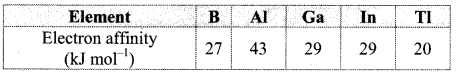Question A.
What is hybridization of Al in AlCl3?
Al is sp2 hybridized in AlCl3.

Question B.
Name a molecule having banana bond.
Diborane (B2H6)7. Draw the structure of the following

Question A.
Orthophosphoric acid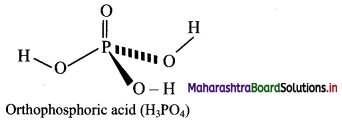Question B.
Resonance structure of nitric acid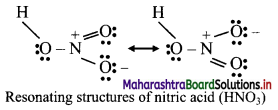8. Find out the difference between

Question A.
Diamond and Graphite
Diamond:

1. It has a three-dimensional network structure.
2. In diamond, each carbon atom is sp3 hybridized.
3. Each carbon atom in diamond is linked to four other carbon atoms.
4. Diamond is poor conductor of electricity due to absence of free electrons.
5. Diamond is the hardest known natural substance.

Graphite:

1. It has a two-dimensional hexagonal layered structure.
2. In graphite, each carbon atom is sp2 hybridized.
3. Each carbon atom in graphite is linked to three other carbon atoms.
4. Graphite is good conductor of electricity due to presence of free electrons in its structure.
5. Graphite is soft and slippery.

Question B.
White phosphorus and Red phosphorus
White phosphorus:

1. It consists of discrete tetrahedral P4 molecules.
2. It is less stable and more reactive.
3. It exhibits chemiluminescence.
4. It is poisonous.

Red phosphorus:

1. It consists chains of P4 molecules linked together by covalent bonds.
2. It is stable and less reactive.
3. It does not exhibit chemiluminescence.
4. It is nonpoisonous.9. What are silicones? Where are they used?
i. a. Silicones are organosilicon polymers having R2SiO (where, R = CH3 or C6H5 group) as a repeating unit held together by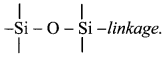b. Since the empirical formula R2SiO (where R = CH3 or C6H5 group) is similar to that of ketones (R2CO), these compounds are named as silicones.

ii. Applications: They are used as

• insulating material for electrical appliances.
• water proofing of fabrics.
• sealant.
• high temperature lubricants.
• for mixing in paints and enamels to make them resistant to high temperature, sunlight and chemicals.

10. Explain the trend in oxidation state of elements from nitrogen to bismuth.

• Group 15 elements have five valence electrons (ns2 np3). Common oxidation states are -3, +3 and +5. The range of oxidation state is from -3 to +5.
• Group 15 elements exhibit positive oxidation states such as +3 and +5. Due to inert pair effect, the stability of +5 oxidation state decreases and +3 oxidation state increases on moving down the group.
• Group 15 elements show tendency to donate electron pairs in -3 oxidation state. This tendency is maximum for nitrogen.
• The group 15 elements achieve +5 oxidation state only through covalent bonding.
e. g. NH3, PH3, ASH3, SbH3, and BiH3 contain 3 covalent bonds. PCl5 and PF5 contain 5 covalent bonds.

11. Give the test that is used to detect borate radical is qualitative analysis.
i. Borax when heated with ethyl alcohol and concentrated H2SO4, produces volatile vapours of triethyl borate, which bum with green edged flame.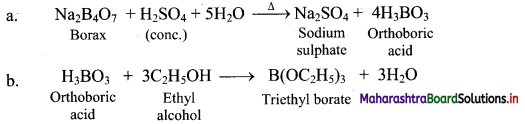ii. The above reaction is Used as a test for the detection and removal of borate radical $$\left(\mathrm{BO}_{3}^{3-}\right)$$ in qualitative analysis.12. Explain structure and bonding of diborane.

• Electronic configuration of boron is 1s2 2s2 2p1. Thus, it has only three valence electrons.
• In diborane, each boron atom is sp3 hybridized. Three of such hybrid orbitals are half filled while the fourth sp3 hybrid orbital remains vacant.
• The two half-filled sp3 hybrid orbitals of each B atom overlap with 1s orbitals of two terminal H atoms and form four B – H covalent bonds. These bonds are also known as two-centred-two-electron (2c-2e) bonds.
• When ‘1s’ orbital of each of the remaining two H atoms simultaneously overlap with half-filled hybrid orbital of one B atom and the vacant hybrid orbital of the other B atom, it produces two three-centred-two- electron bonds (3c-2e) or banana bonds.
• Hydrogen atoms involved in (3c-2e) bonds are the bridging H atoms i.e., H atoms in two B – H – B bonds.
• In diborane, two B atoms and four terminal H atoms lie in one plane, while the two bridging H atoms lie symmetrically above and below this plane.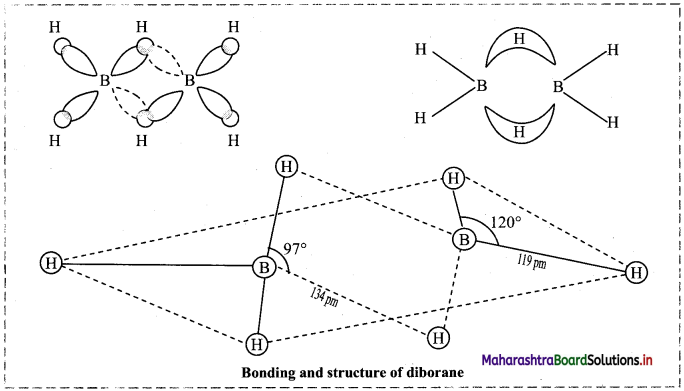13. A compound is prepared from the mineral colemanite by boiling it with a solution of sodium carbonate. It is white crystalline solid and used for inorganic qualitative analysis.

a. Name the compound produced.
b. Write the reaction that explains its formation.
a. Borax
b. Borax is obtained from its mineral colemanite by boiling it with a solution of sodium carbonate.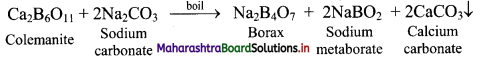14. Ammonia is a good complexing agent. Explain.
i. The lone pair of electrons on nitrogen atom facilitates complexation of ammonia with transition metal ions. Thus, ammonia is a good complexing agent as it forms complex by donating its lone pair of electrons.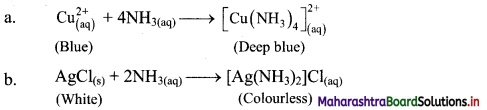ii. This reaction is used for the detection of metal ions such as Cu2+ and Ag+.

15. State true or false. Correct the false statement.

A. The acidic nature of oxides of group 13 increases down the graph.
B. The tendency for catenation is much higher for C than for Si.
A. False
The acidic nature of oxides of group 13 decreases down the group. It changes from acidic through amphoteric to basic.
B. True16. Match the pairs from column A and B.

 Column A Column B i. BCl3 a. Angular molecule ii. SiO2 b. Linear covalent molecule iii. CO2 c. Tetrahedral molecule d. Planar trigonal molecule

i – d,
ii – c,
iii – b

17. Give the reactions supporting basic nature of ammonia.
In the following reactions ammonia reacts with acids to form the corresponding ammonium salts which indicates basic nature of ammonia.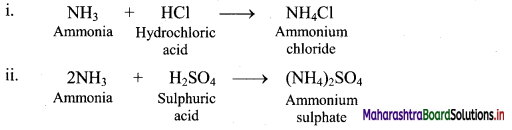18. Shravani was performing inorganic qualitative analysis of a salt. To an aqueous solution of that salt, she added silver nitrate. When a white precipitate was formed. On adding ammonium hydroxide to this, she obtained a clear solution. Comment on her observations and write the chemical reactions involved.
i. When silver nitrate (AgNO3) is added to an aqueous solution of salt sodium chloride (NaCl), a white precipitate of silver chloride (AgCl) is formed.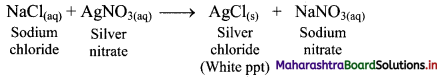ii. On adding ammonium hydroxide (NH4OH) to this, the white precipitate of silver chloride gets dissolved and thus, a clear solution is obtained.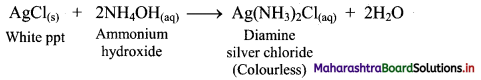11th Chemistry Digest Chapter 9 Elements of Group 13, 14 and 15 Intext Questions and Answers

Can you recall? (Textbook Page No. 123)

Question 1.
If the valence shell electronic configuration of an element is 3s2 3p1, in which block of the periodic table is it placed?
The element having valence shell electronic configuration 3s2 3p1 must be placed in the p-block of the periodic table as its last electron enters in p-subshell (3p).

Can you recall? (Textbook Page No. 127)

Question 1.
What is common between diamond and graphite?
Both diamond and graphite are made up of carbon atoms as they are two allotropes of carbon.

Can you recall? (Textbook Page No. 129)

Question i.
Which element from the following pairs has higher ionization enthalpy?
B and TI, N and Bi
Among B and Tl, boron has higher ionization enthalpy while, among N and Bi, nitrogen has higher ionization enthalpy.

Question ii.
Does boron form covalent compound or ionic?